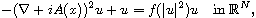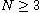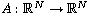Electron. J. Differential Equations, Vol. 2018 (2018), No. 174, pp. 1-21.

### Nontrivial complex solutions for magnetic Schrodinger equations with critical nonlinearities Sara Barile, Giovany M. Figueiredo

Abstract:
Using minimization arguments we establish the existence of a complex solution to the magnetic Schrodinger equationwhere,is the magnetic potential and f satisfies some critical growth assumptions. First we obtain bounds from a real Pohozaev manifold. Then relate them to Sobolev imbedding constants and to the least energy level associated with the real equation in absence of the magnetic field (i.e., with A(x)=0). We also apply the Lions Concentration Compactness Principle to the modula of the minimizing sequences involved.

Submitted September 14, 2017. Published October 22, 2018.
Math Subject Classifications: 35B33, 35J20, 35Q55.
Key Words: Magnetic Schrodinger equations; critical nonlinearities; minimization problem; concentration-compactness methods; Pohozaev manifold.

Show me the PDF file (315 KB), TEX file for this article.Sara Barile Dipartimento di Matematica Università degli Studi di Bari Aldo Moro Via E. Orabona 4, 70125 Bari, Italy email: sara.barile@uniba.it Giovany M. Figueiredo Universidade de Brasilia - UNB Departamento de Matemática Campus Universitário Darcy Ribeiro Brasilia - DF, CEP 70.910-900, Brazil email: giovany@unb.br